Function Repository Resource:

# ArcTanDegree

Compute the inverse tangent of a number and get a result in degrees

Contributed by: Paco Jain (Wolfram Research)
 ResourceFunction["ArcTanDegree"][x] computes the inverse tangent of x in degrees. ResourceFunction["ArcTanDegree"][x,y] computes the inverse tangent of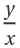in degrees, taking into account which quadrant the point (x,y) is in.

## Details

Mathematical function, suitable for both symbolic and numerical manipulation.
ResourceFunction["ArcTanDegree"][x] always returns a value between -90 and 90 for real x.
For certain special arguments, ResourceFunction["ArcTanDegree"] automatically evaluates to exact values.
ResourceFunction["ArcTanDegree"] can be evaluated to arbitrary numerical precision.

## Examples

### Basic Examples (2)

Compute the inverse tangent of a number:

 In:=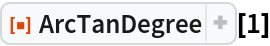Out=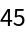Plot over a given range of values:

 In:=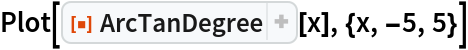Out=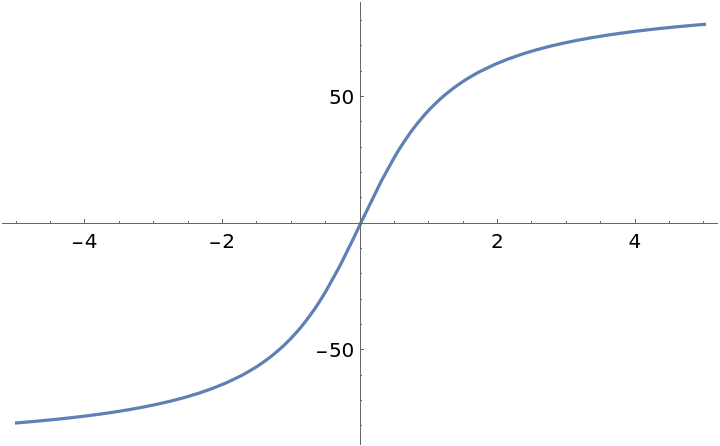ArcTanDegree[x,y] gives the angle of the point {x,y}:

 In:=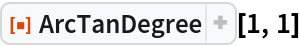Out=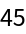In:=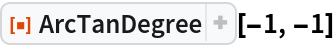Out=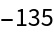### Scope (2)

Evaluate numerically:

 In:=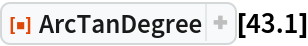Out=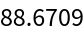In:=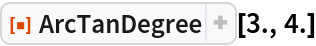Out=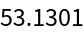Evaluate to high precision:

 In:=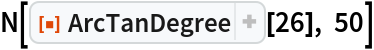Out=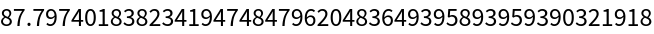In:=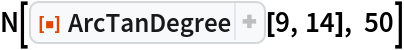Out=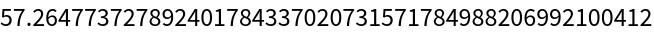### Applications (2)

Find angles of the right triangle with sides 3, 4 and hypotenuse 5:

 In:=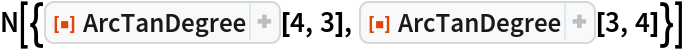Out=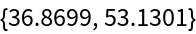They total to 90°:

 In:=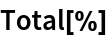Out=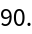## Requirements

Wolfram Language 11.3 (March 2018) or above

## Version History

• 1.1.0 – 13 September 2021
• 1.0.0 – 27 November 2018